##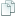Topic: Maths for Videogame Developers (Read 6430 times)previous topic - next topic

0 Members and 1 Guest are viewing this topic.
Maths for Videogame Developers
##### July 12, 2013, 05:46:37 am
I want to get some great codding skills and realize that Indeed maths and physics is very important even when working with very simple 2d games. What kind of maths would you recommend I learn to sharpen my skills:

Radnen already mentioned algebra and trigonometry but could you suggest a more specific list with some of its practical use in a video-game?

Thanks for your absolute enthusiasm hombres.

••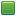• Senior Staff
• Wise Warrior
Re: Maths for Videogame Developers
##### Reply #1 – July 12, 2013, 06:05:37 am
Okay, well for starters Pythagorean theorem is used to find distances. It uses two vectors to find a third one: namely use x and y to find z.

It goes like this in code, to find a distance between a player and an enemy:
Code: (javascript) [Select]
`var dx = player_x - enemy_x;var dy = player_y - enemy_y;var distance = Math.sqrt(dx*dx + dy*dy);Abort("My distance away from the enemy is: "+ distance);`

The above is useful in action games, especially in games with projectiles. You can also use it for volume property on "3D sounds":
Code: (javascript) [Select]
`var dx = source_x - player_x;var dy = source_y - player_y;var distance = Math.sqrt(dx*dx + dy*dy);sound.setVolume((1- (distance / max_distance)) * 255);`

As you get closer to the sound, the sound gets louder.

I'll show more examples tomorrow.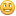If you use code to help you code you can use less code to code. Also, I have approximate knowledge of many things.

Sphere-sfml here
Sphere Studio editor here

Re: Maths for Videogame Developers
##### Reply #2 – July 12, 2013, 09:27:05 am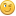I'm definitely going to use this. 3d sound Wow (*_*).

I found this really interesting article on Gamasutra. It really hit home.

Quote

What turns game coders off about mathematics (apart from a difficult writing style) is the apparent lack of relevance to games. This doesn't have to be the case! In this article I will try to show how some simple mathematical ideas are applied to game coding.

• N E O
••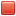Re: Maths for Videogame Developers
##### Reply #3 – July 12, 2013, 04:51:24 pm
Specific examples of maths in game dev:

*   If it's still around somewhere, take a look at my old NRingMenu demo where I recreate the style of menu found in the SNES Seiken Densetsu games (aka "Secret of Mana"); sine and cosine power the circular coordinates and timed rotation animation!
*   Trigonometry is also a major part of 3D engines and "enhanced" isometric mapping like that found in tactics RPG games like Final Fantasy Tactics and Tactics Ogre. I attempted a tech demo a long time ago but I don't know where it is. Look for Beaker's "The Rainis Manuscript" for a better sample of this.

Regarding the Pythagorean distance formula, the one warning about it is that square-root is often one of the more expensive functions a computer can do, so if you've reached a point in game dev where you're looking to speed up code consider using distance squared instead (ie, everything above until the square root).

That Gamasutra article is awesome, BTW.

• Fat Cerberus
••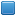• Global Moderator
• miniSphere Developer
Re: Maths for Videogame Developers
##### Reply #4 – July 12, 2013, 05:54:42 pm
@NEO
Is sqrt() really more expensive than squaring?  Mathematically sqrt(x) is the same as x^0.5, the two operations should (in theory) have identical performance assuming both are done in floating point...
Sphere 5.5.1 - miniSphere engine - Cell compiler - SSj debugger

• N E O
••Re: Maths for Videogame Developers
##### Reply #5 – July 12, 2013, 06:15:01 pm
Square can be done as x*x if you ignore use of pow(); sqrt() on computers often involves a Taylor series expansion in the background somehow (or the Babylonian/Newton root approximation method), and a*b multiplication is always faster than that even in this era of dual-, quad-, hexa-, and octo-core CPUs.

• alpha123
•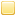Re: Maths for Videogame Developers
##### Reply #6 – July 13, 2013, 01:11:23 am
You'll definitely want to know linear algebra pretty well, it's useful in both 2D and especially 3D games for all sorts of things, in 3D camera manipulation and model orientation is a big one; in 0 A.D. we use a little linear algebra to calculate splash damage.

• Flying Jester
•• TurboSphere Developer
Re: Maths for Videogame Developers
##### Reply #7 – July 13, 2013, 04:44:17 am
Perhaps not really a thing to learn, but to keep in mind: decide way before you start writing code (or perhaps after a failed attempt, before you start a new one) whether you want to measure movement as polar velocities or as a speed and angle (I prefer the latter).

Also, pretty basic trigonometry is very, very useful. In fact, I didn't know the real use of tangent or arctangent until I started coding (where it was exceptionally useful).

For visual effects, Gaussian distribution equations are pretty handy. Once you know what it looks like (and can visualize it being applied to variables), it can help a lot with making more aesthetically appealing effects.

I don't know about older Spidermonkey, but with V8 sqrt() primarily uses lookup tables (same with most trig functions). Similarly, V8 changes 'x/2' to a faster bitshift when possible. On the other hand, it will not apply that to 'x*0.5' (there's a valid reason to do it that way, or so I'm told). The only mathematical optimization advice I have that isn't (or won't be after some trial and error) is to use whole numbers whenever possible (the 2 in 'x/2', as opposed to the 0.5 in 'x*0.5').••• Senior Staff
• Wise Warrior
Re: Maths for Videogame Developers
##### Reply #8 – July 13, 2013, 05:21:58 am
Using Math.atan2 to look-at people and objects in a top-down game. In this demo the cursor will 'look-at' the mouse as you move it around the screen.

Code: (javascript) [Select]
`function TestMouse(){	var done = false;    var arrow = GetSystemArrow();    var a_x = GetScreenWidth() / 2 - arrow.width / 2;    var a_y = GetScreenHeight() / 2 - arrow.height / 2;	var green = CreateColor(0, 255, 0);    while (!done) {        var x = GetMouseX();        var y = GetMouseY();        var dx = x - (a_x + arrow.width / 2);        var dy = y - (a_y + arrow.height / 2);        var angle = Math.atan2(dy, dx);		Point(x, y, green);        arrow.rotateBlit(a_x, a_y, angle);        FlipScreen();		while (AreKeysLeft()) {			if (GetKey() == KEY_ENTER) done = true;		}    }}`
If you use code to help you code you can use less code to code. Also, I have approximate knowledge of many things.

Sphere-sfml here
Sphere Studio editor here

Re: Maths for Videogame Developers
##### Reply #9 – July 13, 2013, 08:13:08 am
The Mersenne Twister
A pseudo random number generator.
One among many things I've been taking time
out to learn for an upcoming part of my project.
(Example from Wiki Article:)

Code: (javascript) [Select]
` // Create a length 624 array to store the state of the generator int[0..623] MT int index = 0  // Initialize the generator from a seed function initialize_generator(int seed) {     index := 0     MT := seed     for i from 1 to 623 { // loop over each other element         MT[i] := last 32 bits of(1812433253 * (MT[i-1] xor (right shift by 30 bits(MT[i-1]))) + i) // 0x6c078965     } }  // Extract a tempered pseudorandom number based on the index-th value, // calling generate_numbers() every 624 numbers function extract_number() {     if index == 0 {         generate_numbers()     }      int y := MT[index]     y := y xor (right shift by 11 bits(y))     y := y xor (left shift by 7 bits(y) and (2636928640)) // 0x9d2c5680     y := y xor (left shift by 15 bits(y) and (4022730752)) // 0xefc60000     y := y xor (right shift by 18 bits(y))     index := (index + 1) mod 624     return y }  // Generate an array of 624 untempered numbers function generate_numbers() {     for i from 0 to 623 {         int y := (MT[i] & 0x80000000)                       // bit 31 (32nd bit) of MT[i]                        + (MT[(i+1) mod 624] & 0x7fffffff)   // bits 0-30 (first 31 bits) of MT[...]         MT[i] := MT[(i + 397) mod 624] xor (right shift by 1 bit(y))         if (y mod 2) != 0 { // y is odd             MT[i] := MT[i] xor (2567483615) // 0x9908b0df         }     } }`

Though, Radnen helped out with this in another thread.He cool.

. . .

And for platforming games: Law of Motion and Inertia

Quote
The first of the these laws is the Law of Inertia, which, to put in a very blunt and non-technical way, states :

* Objects are lazy.

Go get a cup of coffee, or a RockStar energy drink, and think about this for a moment. While you are doing this, notice all the lazy objects around you. Then, come back.

You may have noticed that nothing moved -- unless you moved it. This is half of the Law of Inertia. Surprisingly, the second part is that if an object is in motion, it will keep moving. Here's the Law of Inertia stated more properly :

* An object tend to stay still or keep moving in a straight line if no other forces act on them.

You may have heard that if you hit a golf ball in space that it will travel on forever. This is part of the Law of Inertia. The golf ball will not actually travel forever. It will travel until it finds a force capable of changing its motion. This could be the gravity of a star many billions of miles away, or it could possibly smash into an asteroid. But then, the pieces would travel until there is force to act on them.

https://github.com/kripken/ammo.js/
Linked is a Bullet physics  engine to JavaScript using Emscripten

• Fat Cerberus
••• Global Moderator
• miniSphere Developer
Re: Maths for Videogame Developers
##### Reply #10 – July 13, 2013, 09:55:31 am

The only mathematical optimization advice I have that isn't (or won't be after some trial and error) is to use whole numbers whenever possible (the 2 in 'x/2', as opposed to the 0.5 in 'x*0.5').

Barring the aforementioned special case of bit shift optimization, I don't think this really applies in the general case, since all numbers in JS are floating point.  And even in languages that have a native integer type, from what I've gathered, nowadays, with modern CPUs, trying to do everything in fixed point can actually hurt your performance instead of help.  FP can actually be faster in a lot of cases.
Sphere 5.5.1 - miniSphere engine - Cell compiler - SSj debugger

• alpha123
•Re: Maths for Videogame Developers
##### Reply #11 – July 13, 2013, 12:07:27 pm
Verlet integration is very useful for a number of things, in particular simulating soft-body physics easily.

Barring the aforementioned special case of bit shift optimization, I don't think this really applies in the general case, since all numbers in JS are floating point.

All numbers in JS can be used as double-precision floating point, but the spec doesn't say anything about how they're implemented.Modern JS engines can use a native integer when they can infer that a number is only used as an integer.

• Flying Jester
•• TurboSphere Developer
Re: Maths for Videogame Developers
##### Reply #12 – July 13, 2013, 09:49:43 pm
Almost all modern JS engines try first to put the number into a native integer, then if necessary a 32-bit float (at least V8 has this step), then finally into a fully-compliant 80-bit float.

And in fact, in the V8 high-optimizer (and I assume in some capacity in other engines) these distinctions are very important for optimizing. The high-optimizer (I think it is called Lithium) primarily works to profile functions based on what data types they take, and you can easily force them to 'spin-up' again if you change the types going into functions. And in pure machine code as well, it's harder to pass a lot of FP numbers to a function than a lot of hardware integers.

So it's a good idea to use whole numbers when possible. Maybe not a huge deal, but it's simple enough to do, and doesn't hurt readability.

Floating points can be fast. So can integers, particularly when a lot of modern processors have twice as many integer units as floating point units. Probably, the reason that engines prefer to try and use integers is that it is fast to make a hardware int a floating point, but slow to go the other way.Re: Maths for Videogame Developers
##### Reply #13 – July 25, 2013, 04:45:22 am
Ey, know I'm a bit late, but I think you'll enjoy this gem:

http://www.essentialmath.com/tutorial.htm

Check it out when you're curious.

• DaVince
••Vakinox, that's going to save me some day. I know it. (Bad at maths.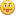)